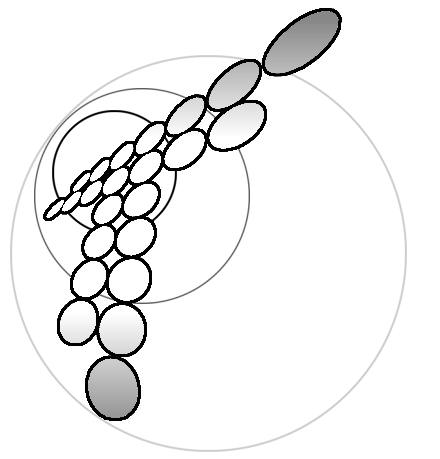# Potential and Charge Distribution in Diffusion Layer for Electrode Process with Subsequent Homogeneous Reaction.

A.V.Sokirko*,Yu.I.Kharkats

A.N.Frumkin Institute of Electrochemistry of Academy of Sciences of Russia, Leninski prosp.31, 117071 Moscow (Russia)

* Dep.of Hydromechanics, The Royal Institute of Technology,10044 Stockholm (Sweden)

It is know that during electric current passing, a space charge region arises in diffusion layer, whose distribution density does not decrease exponentially as it was in diffuse layer, but gradually (1). When the current density tends to its limiting value, the space charge density in the vicinity of the electrode increases abruptly, coinciding in the sign with that for electroactive ions.

Here we present the results of investigation of space charge distribution in diffusion layer for reduction of cations Az+ + z e- -> A0, and parallel oxygen reduction O2 + 2H2O+4e-->4OH- with subsequent recombination reaction in diffusion layer: OH-+H+ ->.H2O. This reaction scheme was analyzed in ref.(2,3), in which the mechanism of migration current exaltation effect in acidic solution was theoretically investigated.

Taking into account that the recombination reaction for water is very fast and equilibrium constant satisfies inequality kÇ1, we can suppose that in the diffusion layer either ions OH- or H+ can exist simultaneously. These approximations make it possible to determine the potential distribution f(x), then using the Poisson equation, to find space charge distribution r=- e f".

The analysis shows that as a result of recombination reaction inside the diffusion layer some interesting peculiarities in space charge distribution arise. at the point of homogeneous reaction localization an abrupt change of sign and absolute value of r takes place. In discussed model due high value of rate constant of recombination reaction its reaction layer had practically zero thickness. In a more general case of moderate reaction rates there must exist some transition region in which space charge varies from positive to negative values. Qualitatively the obtained result can be explained as follows. We can present the plane of recombination reaction localization as some electrode plane at x=q, which is on the one hand brought into contact with H+ ions, and on the other hand with OH- ions. Then in the region q²x²1, where H+ ions exist we have positive space charge distribution as usual in electrolyte. And in the region 0²x²q where OH- exists we have correspondingly negative space charge distribution.

We note in conclusion that the peak in space charge distribution including also a change in the sign of charge was earlier predicted (4,5) for the systems in which besides movable carries of charge also fixed charges exist.

References

(1) V.G.Levich, Physicochemical Hydrodynamics, Prentice-Hall,Inc.,(1962).

(2).A.V.Sokirko, Yu.I Kharkats, .Sov. Electrochemistry, 25,200,(1989).

(3).A.V.Sokirko, Yu.I Kharkats, .Sov. Electrochemistry, 27,667,(1991).

(4).Yu.I Kharkats, .Sov. Electrochemistry, 20,248,(1984).

(3).Yu.Ya.Gurevich,A.V.Noskov, Yu.I Kharkats, .Sov. Electrochemistry, 26,383, (1988).

Skin: